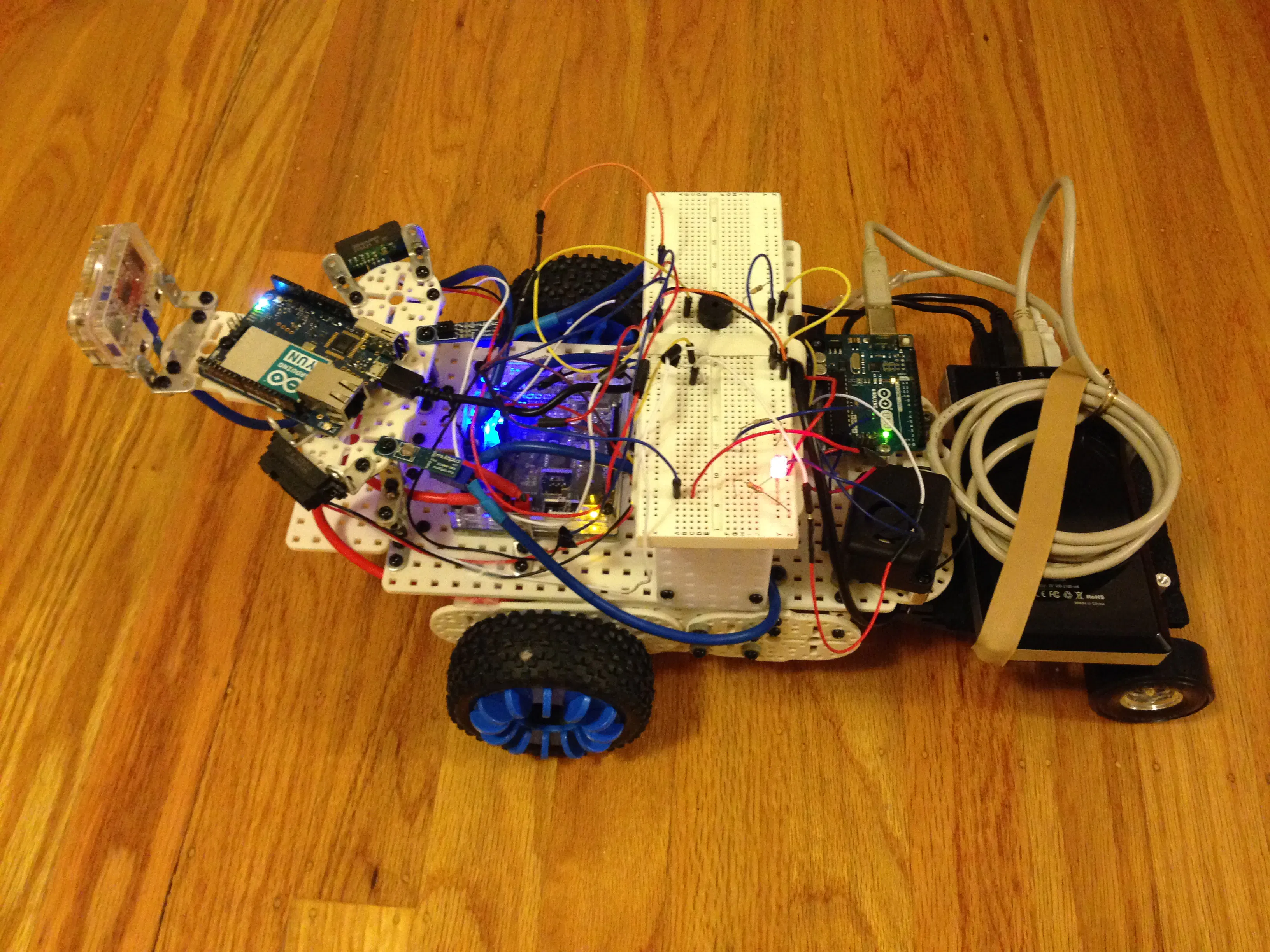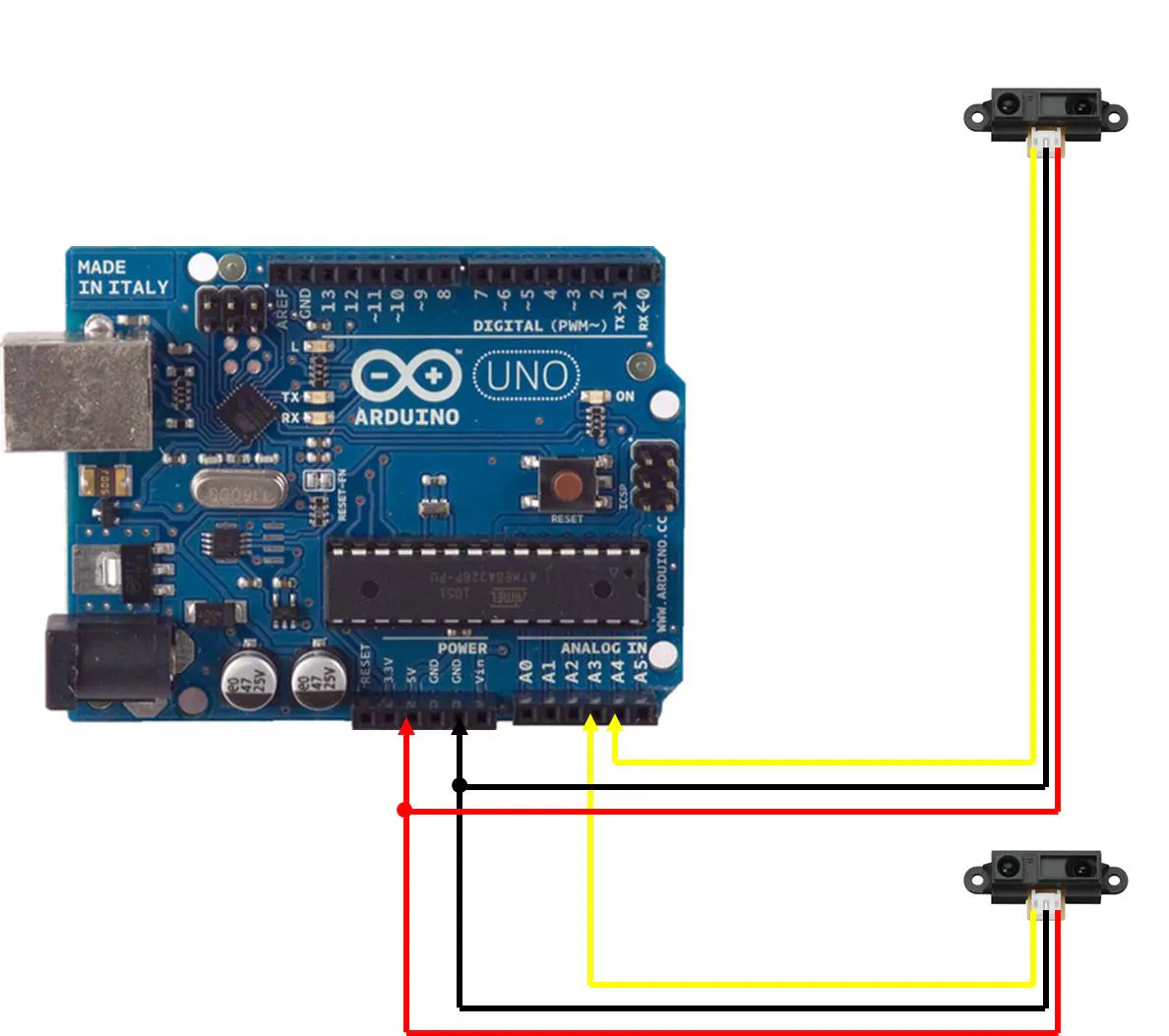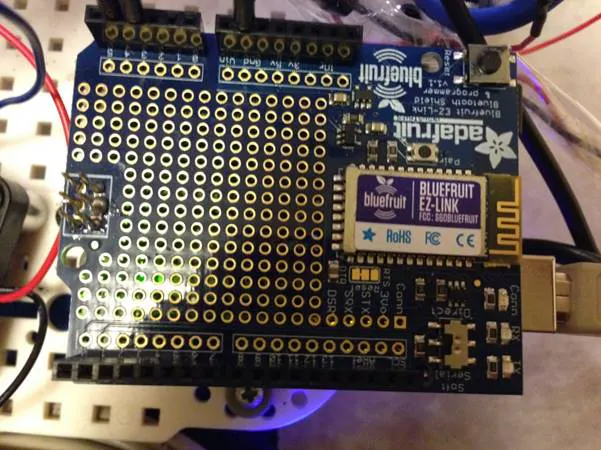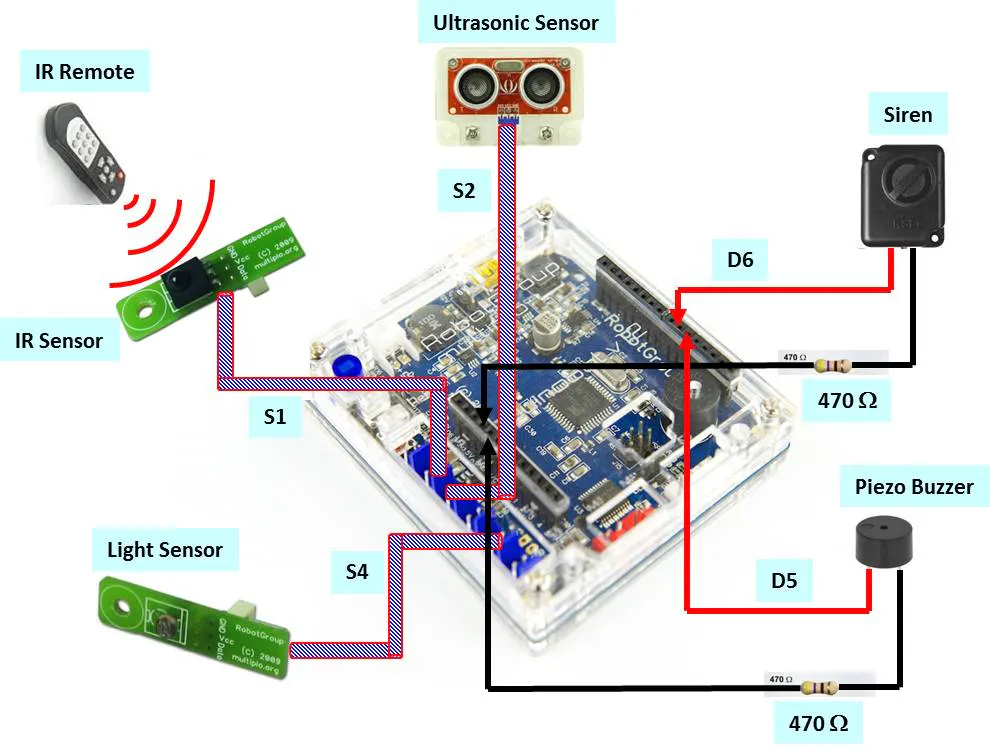# Audible Vision

Duffy the mobile robot moves alongside you connected through a "light leash" and alerts you of objects around the path.

BeginnerFull instructions provided5 hours2,968## Things used in this project

### Hardware componentsArduino UNO
×1Jumper wires (generic)
×1
×1
 470 ohm Resistor (5-pack)
×1
×1×1
×1
 Light Sensor
×1
 Remote IR Sensor
×1
 Siren
×1

### Hand tools and fabrication machinesSoldering iron (generic)

## Schematics

### Fig 1) Setup of IR distance sensors with Arduino Uno

Analog IN Pins #3 and #4 are used to detect objects at left or right of moving Multiplo-Bot. This information is then sent to a Windows 10 Laptop via the Bluetooth communicationThe switch is set to “Direct” mode for Bluetooth communication with the Laptop’s Virtual Shield for Arduino App. The switch is set to “Soft Serial” mode for downloading Arduino code via USB cable.### Fig 3) Setup of Sensors and Audio with Duino-Bot

The Remote IR and Light Sensors are connected to the Analog IN ports S1 and S4, respectively. The Piezo Buzzer and Siren are connected to the Digital OUT pins #5 and #6, respectively.## Code

### Arduino Uno communicating with Virtual Shield for Windows App

Arduino
Arduino IDE code
```/* About Audible Vision:
*  Arduino detects the distance of an object in front of a robot using three different IR sensors
*  Sends this information to a Windows 10 PC, which uses the Virtual Shields for Arduino app to speak the distance
*  The libraries ArduinoJson, Virtual Shield, and Speech belong to the Windows Virtual Shields for Arduino
*    package that contain a text to speech synthesizer.
*
*  Some of the following code is borrowed from: http://luckylarry.co.uk/category/programming-tutorials/arduino-programming/
*
*  February 2016
*  Written by Daniel Martin
*/

//include all libraries
#include <Average.h>

#include <ArduinoJson.h>

#include <VirtualShield.h>

#include <Text.h>

#include <Speech.h>

// Instantiate the shields.
VirtualShield shield;

Speech speech = Speech(shield);

int pin2 = 4;     //initializes pin1 as analog pin A1 on the Uno for the left sensor
//int pin2 = 2;     //initializes pin2 as analog pin A2 on the Uno for the middle sensor
//int pin3 = 3;     //initializes pin3 as analog pin A3 on the Uno for the right sensor

void setup() {
Serial.begin(9600);

// Begin the shield communication
shield.begin(9600);

}

void loop() {

Detect1();    //handles the voltage read from pin 1
//Detect2();    //handles the voltage read from pin 2
//Detect3();    //handles the voltage read from pin 3

}

//Detect1 reads in the voltage of pin 1 and converts it to units of cm.
void Detect1()
{
float volts1 = analogRead(pin2)*0.0048828125;   // value from sensor * (5/1024) - if running 3.3.volts then change 5 to 3.3
/*if(volts1 < 0.4)
{
volts1 = 0.4;
}
else if(volts1 > 5)
{
volts1 = 5;
}*/
float distance = 65*pow(volts1, -1.10);          // worked out from graph 65 = theretical distance / (1/Volts)S - luckylarry.co.uk
float AA = 0.00000008*pow(distance, 4);
float BB = .00003*pow(distance, 3);
float CC = .005*pow(distance, 2);
float DD = 0.11*distance;
float EE = 4.9093;
float FINAL = AA-BB+CC+DD+EE;                //the final distance, unrounded, is the sum of the terms in the quartic equation
int fin = FINAL;                             //the final distance, rounded to the nearest cm.

///*Serial.print("Object ");
//Serial.println(fin);
String Fin = String(fin);
speech.speak("Object " + Fin + " centimeters to your right");
delay(5000);
}

//Detect2 reads in the voltage of pin 2 and converts it to units of cm.
/*void Detect2()
{
float volts2 = analogRead(pin2)*0.0048828125;   // value from sensor * (5/1024) - if running 3.3.volts then change 5 to 3.3
if(volts2 < 0.4)
{
volts2 = 0.4;
}
else if(volts2 > 5)
{
volts2 = 5;
}
float distance = 65*pow(volts2, -1.10);          // worked out from graph 65 = theretical distance / (1/Volts)S - luckylarry.co.uk
float AA = 0.00000008*pow(distance, 4);
float BB = .00003*pow(distance, 3);
float CC = .005*pow(distance, 2);
float DD = 0.11*distance;
float EE = 4.9093;
float FINAL = AA-BB+CC+DD+EE;                //the final distance, unrounded, is the sum of the terms in the quartic equation
int fin = FINAL;                             //the final distance, rounded to the nearest cm.

Serial.print("Object ");
Serial.print(fin);
Serial.println(" centimeters in front of you");

delay(2000);

}

void Detect3()
{
float volts3 = analogRead(pin3)*0.0048828125;   // value from sensor * (5/1024) - if running 3.3.volts then change 5 to 3.3
if(volts3 < 0.4)
{
volts3 = 0.4;
}
else if(volts3 > 5)
{
volts3 = 5;
}
float distance = 65*pow(volts3, -1.10);          // worked out from graph 65 = theretical distance / (1/Volts)S - luckylarry.co.uk
float AA = 0.00000008*pow(distance, 4);
float BB = .00003*pow(distance, 3);
float CC = .005*pow(distance, 2);
float DD = 0.11*distance;
float EE = 4.9093;
float FINAL = AA-BB+CC+DD+EE;                //the final distance, unrounded, is the sum of the terms in the quartic equation
int fin = FINAL;                             //the final distance, rounded to the nearest cm.

Serial.print("Object ");
Serial.print(fin);
delay(2000);

}*/
```

### Duffy responding to Light leash

Arduino
DuinoPack IDE code
```#include <IRremote.h>

int RECV_PIN = 15;

IRrecv irrecv(RECV_PIN);

decode_results results;

int photopin = 18;
int sirenpin = 6;
int directioncheck = 1;

const int pingPin = 16;

void setup()
{
Serial.begin(9600);
pinMode(sirenpin, OUTPUT);
//motor0.setClockwise(false);
}

void loop()
{
// establish variables for duration of the ping,
// and the distance result in inches and centimeters:
long duration, inches, cm;

// The PING))) is triggered by a HIGH pulse of 2 or more microseconds.
// Give a short LOW pulse beforehand to ensure a clean HIGH pulse:
pinMode(pingPin, OUTPUT);
digitalWrite(pingPin, LOW);
delayMicroseconds(2);
digitalWrite(pingPin, HIGH);
delayMicroseconds(5);
digitalWrite(pingPin, LOW);

// The same pin is used to read the signal from the PING))): a HIGH
// pulse whose duration is the time (in microseconds) from the sending
// of the ping to the reception of its echo off of an object.
pinMode(pingPin, INPUT);
duration = pulseIn(pingPin, HIGH);

// convert the time into a distance
inches = microsecondsToInches(duration);
cm = microsecondsToCentimeters(duration);

//Serial.print(inches);
//Serial.print("in, ");
//Serial.println(cm);
//delay(500);
/* Serial.print("cm");
Serial.println();*/

delay(50);

//Serial.println(lightVolts);

if (lightVolts >= 400 && directioncheck == 1 && cm > 35)
{
FWD(20);
}
else if (lightVolts >= 400 && directioncheck == -1)
{
BACK(20);
}
else if (lightVolts <= 400 && cm > 35)
{
BRAKE(20);
}
else if(directioncheck == 1 && cm < 35)
{
//motor0.setSpeed(0);
//motor1.setSpeed(0);
tone(5, 2000, 500);
BRAKE(1000);

}

if (irrecv.decode(&results)) {

digitalWrite(sirenpin, HIGH);

irrecv.resume(); // Receive the next value
//motor0.setSpeed(50);
//motor1.setSpeed(-54);

if((results.value == 32) || (results.value == 2080) || (results.value == 16) || (results.value == 2064)){
BRAKE(3000);
directioncheck = 1;
}
else if ((results.value == 33) || (results.value == 2081) || (results.value == 17) || (results.value == 2065)){
BRAKE(3000);
directioncheck = -1;
}

//delay(500);
digitalWrite(sirenpin, LOW);

}

}

void FWD(int time)
{
motor0.setSpeed(50.0);
motor1.setSpeed(-53.0);
delay(time);
}

void BACK(int time)
{
motor0.setSpeed(-50.0);
motor1.setSpeed(53.0);
delay(time);
}

void BRAKE(int time)
{
motor0.brake();
motor1.brake();

delay(time);
}

long microsecondsToInches(long microseconds)
{
// According to Parallax's datasheet for the PING))), there are
// 73.746 microseconds per inch (i.e. sound travels at 1130 feet per
// second).  This gives the distance travelled by the ping, outbound
// and return, so we divide by 2 to get the distance of the obstacle.
// See: http://www.parallax.com/dl/docs/prod/acc/28015-PING-v1.3.pdf
return microseconds / 74 / 2;
}

long microsecondsToCentimeters(long microseconds)
{
// The speed of sound is 340 m/s or 29 microseconds per centimeter.
// The ping travels out and back, so to find the distance of the
// object we take half of the distance travelled.
return microseconds / 29 / 2;
}
```

## Credits

### Daniel Martin

0 projects • 2 followers
I am studying EE in UC Davis, and interested in tinkering with Arduino and Pi. Some of my Maker projects are at piduino.weebly.com